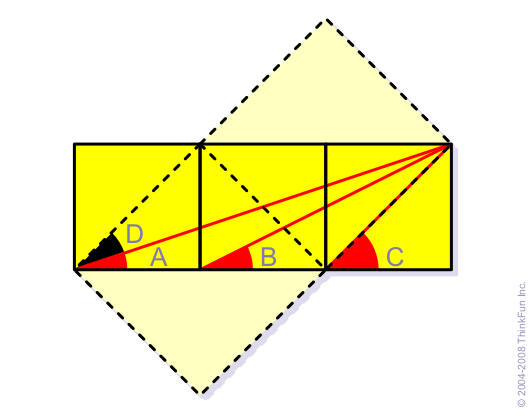Trivia

Subject:
Another proof/demonstration *PIC*
Response To:

Alex Y
Draw two more squares as in the drawing below.Note that angle A plus the new angle D equals angle C, since they both equal the angle (45*) between a side and a diagonal of one of the original squares.

A+D=C

D is equal to B, since they both are angles between the long side and diagonal of a 1x2 rectangle.

Substitute B for D in the previous equation,

A+B=C

qed